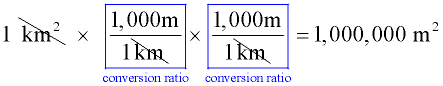Conversions Between Systems MathBitsNotebook.com Terms of Use   Contact Person: Donna Roberts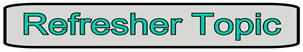Metric to English English to Metric Length: 1millimeter (m) = 0.04 inches (in) 1 centimeter (cm) = 0.39 inches (in) 1 meter (m) = 39.37 inches = 3.28 feet (ft) 1 meter (m) = 1.09 yard (yd) 1 kilometer (km) = 0.62 miles (mi) Length: 1 inch (in) = 2.54 centimeters (cm) 1 foot (ft) = 30.48 cm = 0.305 meters (m) 1 yard (yd) = 0.914 meters (m) 1 mile (mi) = 1.609 kilometer (km) Weight: 1 gram (g) = 0.035 ounces (oz) 1 liter (l) = 1.057 quart (qt) Weight: 1 ounces (oz) = 28.350 grams (g) 1 pound (lb) = 0.453 kilograms (kg) Capacity: 1 milliliter (ml) = 0.2 teaspoon (tsp) 1 liter (l) = 1.057 quart (qt) Capacity: 1 teaspoon (tsp) = 5 milliliters (ml) 1 cup (c) = 236 milliliters (ml) 1 quart (qt) = 0.9461 liter (l) 1 gallon (gal) = 3.785 liter (l)Dimensional Analysis and Conversion Ratios:
It is possible to make simple unit conversions mentally or with a simple one-step multiplication or division. There are, however, more complex conversions which require several conversions between numerous units.

The process of Dimensional Analysis (also called the Unit Factor Method) is a mathematical method that uses the fact that any number or expression can be multiplied by "one" without changing its value. It allows you to convert units by multiplying the old measurement by one (or more) forms of the number 1. While the multiplication by 1 does not change the value of the measurement, it does change the measurement's units.

A conversion ratio (or unit factor) is a ratio equal to one. This ratio carries the names of the units to be used in the conversion. It can be used for conversions within the English and Metric Systems, as well as for conversions between the systems. The conversion ratio is based upon the concept of equivalent values. In the example below, one foot is substituted for its equivalent measure of 12 inches.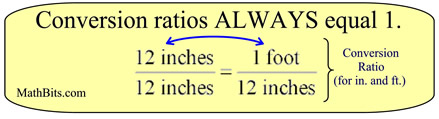The main idea in Dimensional Analysis is to create a conversion ratio (unit factor) which has the units you want in the numerator and the units you already have in the denominator. It may be necessary to multiply by more than one conversion ratio in more difficult problems. Remember that you are setting up for one, or more, of the units to cancel until only the desired units remain. ALL conversion ratios (unit factors) must equal one!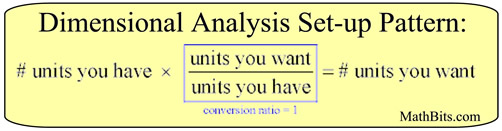Let's try some examples to see how the process works.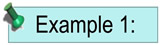(simple problem)
Convert 4.5 kilometers to millimeters.

1 km = 1,000,000 mm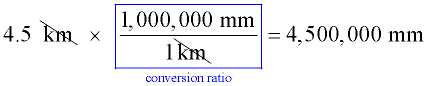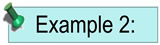(multiple factors)
Convert 65 miles per hour to feet per second.

Notice the need for 3 conversion ratios (all of which equal 1).
All units cancel out except for the desired units.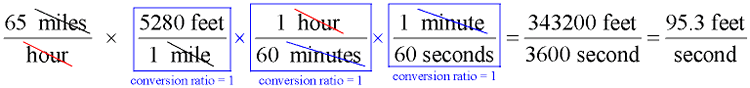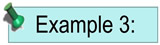(between systems)
Convert 12 yards to centimeters.

Notice the need for 2 conversion ratios (both of which equal 1).
All units cancel out except for the desired units.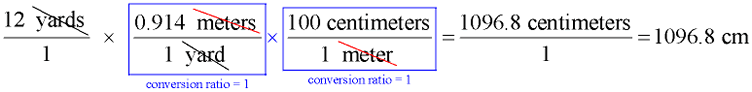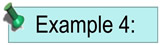(square units)
Convert 1 km2 to m2.